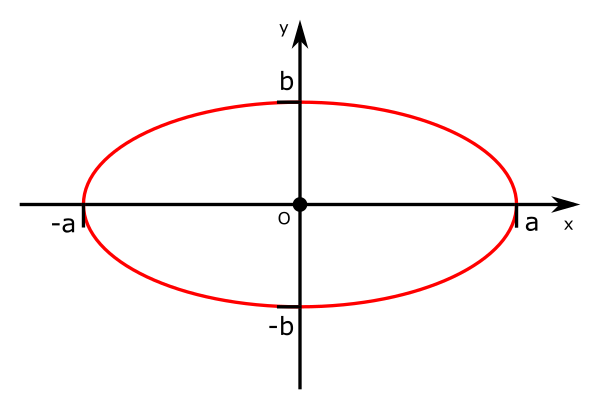# Cartesian equation of ellipse

By Martin McBride, 2020-09-14
Tags: ellipse - cartesian equation
Categories: coordinate systems pure mathematicsThe Cartesian equation of an ellipse is:

$$\frac{x^2}{a^2} + \frac{y^2}{b^2} = 1$$## Understanding the equation

Starting from the parametric equations:

\begin{align} x = a \cos{t}\newline y = b \sin{t} \end{align}

These can be rearranged to give:

\begin{align} \cos{t} = \frac{x}{a}\newline \sin{t} = \frac{y}{b} \end{align}

From Pythagoras we know that:

$$\cos^2{t} + \sin^2{t} = 1$$

Substituting for $\cos{t}$ and $\sin{t}$ gives

\begin{align} \left(\frac{x}{a}\right)^2 + \left(\frac{y}{b}\right)^2 = 1\newline \frac{x^2}{a^2} + \frac{y^2}{b^2} = 1 \end{align}

## Intersection with x and y axes

We can check that this equation correctly predicts where the curve intersects the x and y axes. For the y axis, $x = 0$:

\begin{align} \frac{y^2}{b^2} = 1\newline y^2 = b^2\newline y = b \end{align}

Which is correct, the curve intersect the y axis at $y = b$ and $y = -b$.

For the x axis, $y = 0$:

\begin{align} \frac{x^2}{a^2} = 1\newline x^2 = a^2\newline x = a \end{align}

Which is also correct, the curve intersect the x axis at $x = a$ and $x = -a$.